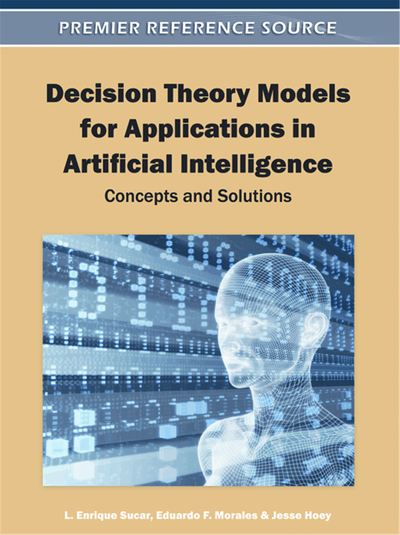# An Introduction to Reinforcement Learning

Eduardo F. Morales (National Institute of Astrophysics, Optics and Electronics, México) and Julio H. Zaragoza (National Institute of Astrophysics, Optics and Electronics, México)
Copyright: © 2012 |Pages: 18
DOI: 10.4018/978-1-60960-165-2.ch004

## Abstract

This chapter provides a concise introduction to Reinforcement Learning (RL) from a machine learning perspective. It provides the required background to understand the chapters related to RL in this book. It makes no assumption on previous knowledge in this research area and includes short descriptions of some of the latest trends, which are normally excluded from other introductions or overviews on RL. The chapter provides more emphasis on the general conceptual framework and ideas of RL rather than on presenting a rigorous mathematical discussion that may require a great deal of effort by the reader. The first section provides a general introduction to the area. The following section describes the most common solution techniques. In the third section, some of the most recent techniques proposed to deal with large search spaces are described. Finally, the last section provides some final remarks and current research challenges in RL.
Chapter Preview
Top

## Introduction

Reinforcement Learning (RL) has become one of the most active research areas in Machine Learning1. In general terms, its main objective is to learn how to map states to actions while maximizing a reward signal. In reinforcement learning an autonomous agent follows a trial-and-error process to learn the optimal action to perform in each state in order to reach its goals. The agent chooses an action in each state, which may take the agent to a new state, and receives a reward. By repeating this process, the agent eventually learns which is the best action to perform to obtain the maximum expected accumulated reward. In general, in each iteration (see Figure 1), the agent perceives its current state (sS), selects an action (aA), possibly changing its state, and receives a reward signal (rR). In this process, the agent needs to obtain useful experiences regarding states, actions, state transitions and rewards to act optimally and the evaluation of the system occurs concurrently with the learning process.

This approach appeals to many researchers because if they want to teach an agent how to perform a task, instead of programming it, which may be a difficult and time-consuming process, they only need, in principle, to let the agent learn how to do it by interacting with the environment.

To illustrate this learning process, suppose that we want a mobile robot to learn how to reach a particular destination in an indoor environment. We can characterize this navigation problem as a RL problem. The states can be defined in terms of the information provided by the sensors of the robot, for instance, if there is an obstacle in front of the robot or not. We can have a finite set of actions per state, such as go-forward, go-backward, go-left and go-right, and the goal may be to go to a particular place (see Figure 2). Each time the robot executes an action there is some uncertainty on the actual next state as the wheels of the robot often slip on the ground or one wheel may turn faster than another, leaving the robot in a possibly different expected state. Upon reaching a goal state, the robot receives a positive reward and similarly receives negative regards in undesirable states. The robot must choose its actions in order to increase in the long turn the total accumulated rewards. By following a trial and error process the robot learns after several trials which is the best action to perform on each state to reach the destination point and obtain the maximum expected accumulated reward.

Figure 2.

Reinforcement Learning process with a mobile robot where the robot may have different possible actions per state and the goal is to learn how to go to a particular destination while receiving the maximum total expected reward

Deciding which action to take in each state is a sequential decision process. In general, we have a non-deterministic environment (the same action in the same state can produce different results). However, it is assumed to be stationary (i.e., the transition probabilities do not change over time). This sequential decision process can be characterized by a Markov Decision Process or MDP. As described in Chapter 3, an MDP, M=<S,A,P,R>, can be described as follows:

• A finite set of states (S)

• A finite set of actions (As) per state (s)

• A transition function P that represent the probability of reaching state s'∈S given an action aA taken at state sS:P(s'|s,a).

## Complete Chapter List

Search this Book:
Reset